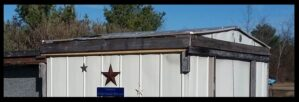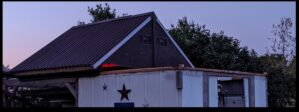The original metal roof of the SCGO observatory has blown off twice in very high winds, once by the remnants of a hurricane and the other by a blizzard. It was the original metal low slope roof that came with the 8’x10′ metal garden shed. Since the second flyaway caused it so much damage, a whole new roof had to be constructed. Never having built a roof before, we laid it out on the ground, intending to give it a higher slope to allow the snow that accumulated on the older low slope roof, to slide off and not be an issue.

In the end, a run of 5.5′ and rise of 4′ was much much too steep. The structure towers 11′ high and maintenance is difficult that high up. Next time it will be a smaller rise!The roof is a little higher on wheels and was difficult to roll off and on. Initially we had three wheels on each side, but thinking that there was too much friction on each wheel axle, we added more wheels. Now there are 7 wheels on each side.
Another question was, were the wheels rated for the roof weight? The issue there was, how much did the assembled-in-place roof actually weigh? Unlike the old roof, this one could not be raised even a little by one person on a corner.

The only realistic method was to estimate the weight based on the list of materials and to weigh samples.
So.. a typical 2x4x8 is 10 lbs, a 1×4 is 6 lbs, the ondura roof segments are 20lbs, a 4’x8′ section of 1/2″ plywood is 40lbs, the wheels 1 lb each.

The base structure was made from 2x4s x10+10+10+10+8+8 = 52 linear feet.
The roof trusses were also 2x4s 7x5x2=70 linear feet
Roof nailers were 1x4s 5x8x2=80 linear feet
eave ends were 1×4 8×2=16 linear feet
1/2 plywood at 40ft^2
14 wheels@ 1lb = 14 lbs

So.. bringing this all together
subtotal 1x4s= 8x5x2 = 80 +16 ends /8*6= 72 lbs
subtotal 2x4s=52+70+4+8=134ft 17*10=170 lbs
subtotal plywood 40ft^2 = 50 lbs
subtotal roof sections 5*20= 100 lbs
subtotal wheels 14*1= 14 lbs
total approximate roof weight = 406 lbs

Assume weight evenly divided over 14 wheels = 406/14=29 lbs each. The wheels are rated for at least 100lbs each.

So the force needed to move the roof is most likely not coming from friction in the wheel axles.
That moves us to the interaction of the wheels and the surface they roll on, which is soft lumber… a topic for next time.